### Addition of Two 3-digit number with Regrouping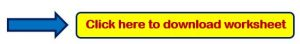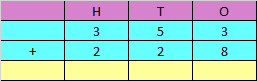Explanation:

Step 1: Adding the digits at one’s place,

3 + 8 = 11

Since the number is more than 9, we need to carry over 1 to the tens place.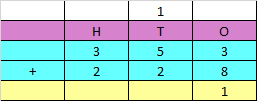Step 2: Adding the digits at tens place and the carry of 1,

5 + 2 + 1 = 8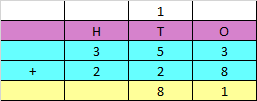Step 3: Adding the digits at hundred’s place,

3 + 2 = 5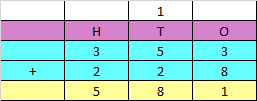Hence, the sum of the given numbers is 581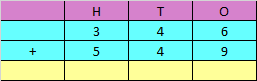Explanation:

Step 1: Adding the digits at one’s place,

6 + 9 = 15

Since the number is more than 9, we need to carry over 1 to the tens place.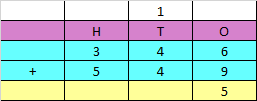Step 2: Adding the digits at tens place and the carry of 1,

4 + 4 + 1 = 9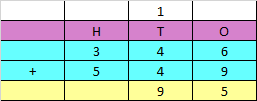Step 3: Adding the digits at hundreds place

3 + 5 = 8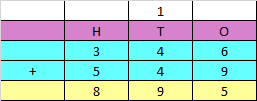Hence, the sum of the given numbers is 895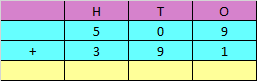Explanation:

Step 1: Adding the digits at one’s place,

9 + 1 = 10

Since the number is more than 9, we need to carry over 1 to the tens place.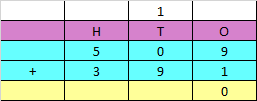Step 2: Adding the digits at tens place and the carry of 1,

0 + 9 + 1 = 10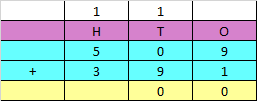Step 3: Adding the digits at hundreds place and the carry of 1,

5 + 3 + 1 = 9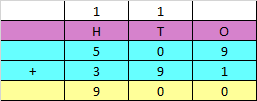Hence, the sum of the given numbers is 900